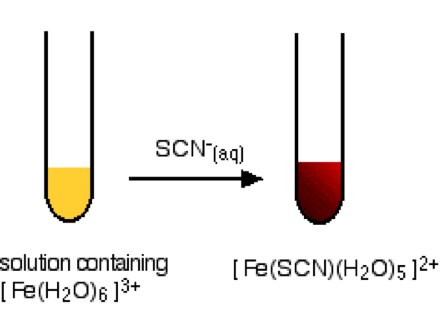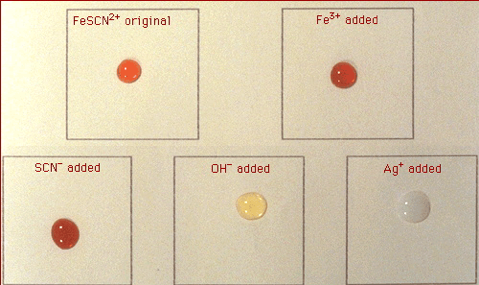# Iron(III) Thiocyanate Equilibrium

This is part of the HSC Chemistry course under the topic Factors Affecting Equilibrium

### HSC Chemistry Syllabus

• Conduct practical investigations to analyse the reversibility of chemical reactions, for example:

– iron (III) nitrate and potassium thiocyanate

• Investigate the effects of temperature, concentration, volume and/or pressure on a system at equilibrium and explain how Le Chatelier’s principle can be used to predict such effects, for example:

– iron(III) thiocyanate and varying concentration of ions (ACSCH095)

### How does the Iron(III) Nitrate & Potassium Thiocyanate equilibrium work?

In this video, we will be exploring what the mechanisms of the iron(III) nitrate and potassium thiocyanate equilibrium are.

### Iron(III) Nitrate Equilibrium

• When iron (III) nitrate and potassium thiocyanate are mixed in solution, an equilibrium mixture of Fe3+, SCN- and the complex ion FeSCN2+ is formed:

$${Fe{(NO_3)}_3}_{(aq)} + \; KSCN_{(aq)} \rightleftharpoons \; {FeSCN^{2+}}_{(aq)} + \; KNO_{3(aq)} \;\;\; \Delta{H}<0$$

• Net ionic equation:

$${Fe^{3+}}_{(aq)} + \; {SCN^-}_{(aq)} \rightleftharpoons \; {FeSCN^{2+}}_{(aq)} \;\;\; \Delta{H}<0$$Figure: a similar reaction that demonstrates the colour change of Fe3+’s conversion to Fe(SCN)2+

• Fe3+ is yellow and FeSCN2+ is blood red.
• Potassium ion and nitrate ion are spectator ions and are not part of the chemical equilibrium.
• Pressure and volume do not affect this reaction as there are no gaseous reactants nor products.

### Concentration• Tube A is a reference tube – used for comparison purposes.
• Tube B: Addition of Na2HPO4: hydrogen phosphate ions react with Fe3+ to form complex ions:

$${Fe^{3+}}_{(aq)} + \; {{HPO_4}^{2-}}_{(aq)} \rightarrow \; {{FeHPO_4}^+}_{(aq)}$$

This reduces [Fe3+] which in turn increases the rate of backward reaction and shifts the chemical equilibrium to the left. Solution turns less blood red, paler yellow or colourless.

• Tube C: Addition of KSCN crystals increases concentration of reactants of forward reaction. This increases the rate of forward reaction and shifts the equilibrium to the right. Solution turns more blood red

• Tube D: Addition of Fe(NO3)3 increases the concentration of reactants available for the forward reaction. This will initially make the solution more yellow as [Fe3+] increases. However, by Le Chatelier’s principle, this change in turn increases the rate of forward reaction and shifts the equilibrium to the right. Solution turns more blood red.

• Addition of SnCl2 (or any form of Sn2+) reduces Fe3+ to Fe2+. Reduction of Fe3+ is possible because Sn2+ is a stronger reductant.

$$Sn^{2+} + 2Fe^{3+} \rightarrow Sn^{4+} + 2Fe^{2+}$$

Reduction decreases [Fe3+] which in turn increases the rate of reverse reaction and shifts the chemical equilibrium to the left. Solution turns less red and more yellow.Figure: colour analysis of Fe3+, SCN- and the complex ion FeSCN2+ equilibrium.

• Adding silver nitrate (AgNO3): Ag+ forms white precipitate with SCN- which decreases [SCN-]:
$${Ag^+}_{(aq)} + {SCN^-}_{(aq)} \rightarrow AgSCN_{(s)}$$

This increases the rate of backward reaction and shifts the chemical equilibrium to the left. Solution turns cloudy white. Precipitate will settle to the bottom of the tube if left undisturbed for a long time.

### Temperature

• The formation of iron (III) thiocyanate complex ion is an exothermic reaction. This means the forward reaction is favoured at a low temperature.Figure: effect of temperature on the dynamic equilibrium of iron thiocyanate solution.

• When the solution is placed in ice-cold water, more iron thiocyanate ion is formed. This causes the solution to appear more blood red.

As the temperature of water increases, the reverse reaction becomes more favoured. This causes the solution to appear less blood red and start to adopt a yellow colour.

Previous section:  Practical Investigations of Le Chatelier's Principle (Cobalt (II) Chloride Equilibrium)

Next section: Practical Investigations of Le Chatelier's Principle (Nitrogen Dioxide and Dinitrogen Tetroxide Equilibrium)